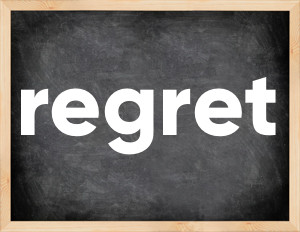# Regret past tenseThe English verb 'regret' is pronounced as [rɪˈɡret].
Related to: regular verbs.
3 forms of verb regret: Infinitive (regret), Past Simple - (regretted), Past Participle - (regretted).

## Here are the past tense forms of the verb regret

👉 Forms of verb regret in future and past simple and past participle.
❓ What is the past tense of regret.

## Regret: Past, Present, and Participle Forms

Base Form Past Simple Past Participle
regret [rɪˈɡret]

regretted [rɪˈɡretɪd]

regretted [rɪˈɡretɪd]

## What are the 2nd and 3rd forms of the verb regret?

🎓 What are the past simple, future simple, present perfect, past perfect, and future perfect forms of the base form (infinitive) 'regret'?

### Learn the three forms of the English verb 'regret'

• the first form (V1) is 'regret' used in present simple and future simple tenses.
• the second form (V2) is 'regretted' used in past simple tense.
• the third form (V3) is 'regretted' used in present perfect and past perfect tenses.

## What are the past tense and past participle of regret?

The past tense and past participle of regret are: regret in past simple is regretted, and past participle is regretted.

### What is the past tense of regret?

The past tense of the verb "regret" is "regretted", and the past participle is "regretted".

### Verb Tenses

Past simple — regret in past simple regretted (V2).
Future simple — regret in future simple is regret (will + V1).
Present Perfect — regret in present perfect tense is regretted (have/has + V3).
Past Perfect — regret in past perfect tense is regretted (had + V3).

### regret regular or irregular verb?

👉 Is 'regret' a regular or irregular verb? The verb 'regret' is regular verb.

## Examples of Verb regret in Sentences

•   Paul regretted that we are gone (Past Simple)
•   The committee has regretted the burning of the mosque (Present Perfect)
•   I will soon regret that I am always in a hurry. (Future Simple)
•   I have never regretted the decision to quit my job. (Present Perfect)
•   Everyone regretted that he was fired. (Past Simple)
•   Subsequently, he regretted that in his youth he had been so incontinent. (Past Simple)
•   They regretted closing the kindergarten. (Past Simple)
•   They subsequently regretted this decision. (Past Simple)
•   He has regretted the disappearance of the Greek language from the school curriculum. (Present Perfect)
•   She regretted what she said as soon as those words escaped her lips. (Past Simple)

Along with regret, words are popular backslide and achieve.

Verbs by letter: , , , , , , , , , , , , , , , , , , , , , , , , .